## Improve Your Nowcasting in SAS Visual Forecasting Using Real Time Data (by Tammy Jackson)

Started ‎12-14-2022 by
Modified ‎12-14-2022 by
Views 533

This blog is based entirely on the demonstration and code written by @TammyJackson , SAS Principal Research Statistician Developer. Special thanks also to Javier Delgado and Thiago Quirino for developing the SAS EXTLANG package and some of the code shown here.

The abundance of real-time and near-real-time data available on the internet has created an amazing opportunity for forecasters to dramatically improve nowcasts. Did you know that data collected in real time can be used to produce high quality nowcasts directly within SAS Visual Forecasting? That’s right, the EXTLANG package lets us pull data from the internet directly into our PROC TSMODEL process.

Background

Tammy Jackson was initially inspired by a presentation at the Women in Statistics and Data Science Conference in 2019 by Samantha Winder, Emilia Lia and Spencer Wood from the University of Washington. In that presentation, Winder et al. compared visitor counts in state and national parks to social media posts including Flickr, Instagram, Twitter. As you can see below, the graphs comparing the seasonal trends from National Park Service surveyed user days (e.g., from trail cams) to the seasonal trends from geolocated Flickr photograph user days correlate very nicely.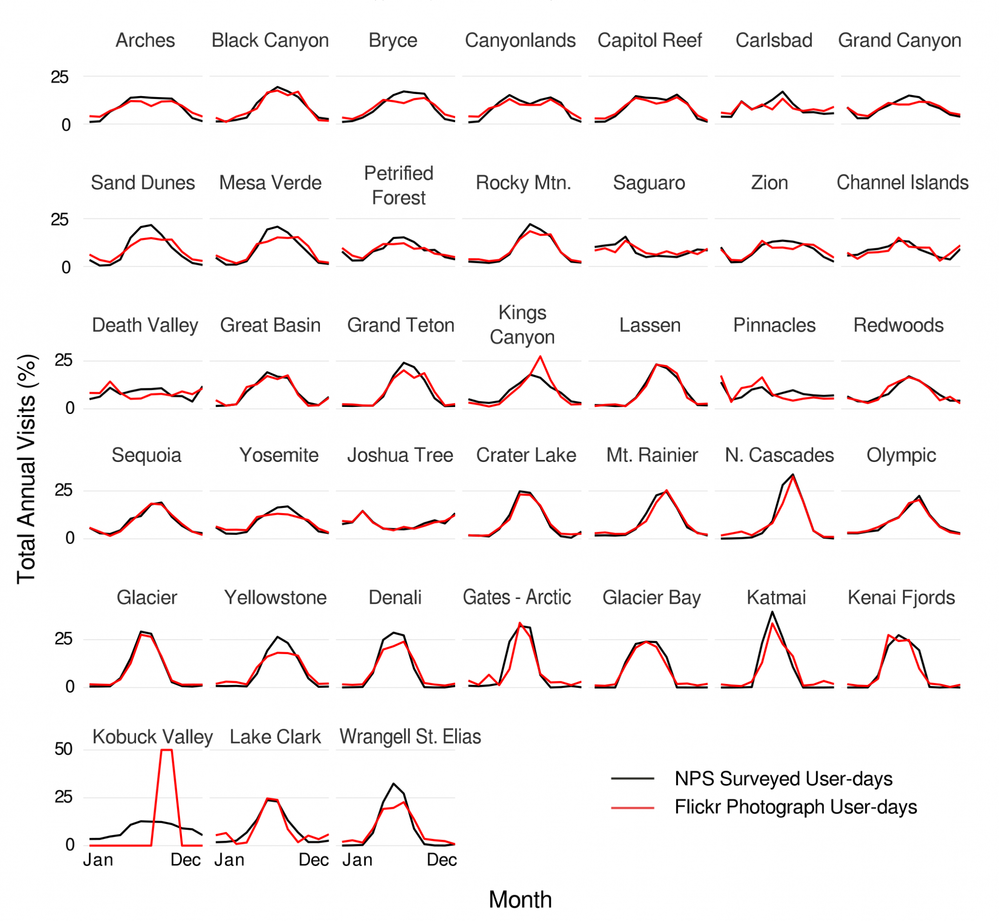Sessions et al 2016 as reported in Winder et al 2019[

Winder et al. successfully came up with a highly accurate model based solely on items they could collect online; it did not use any on site information (trail cams, etc.). Below see the modeled (line) versus observed (dashed line) visitation at an out-of-sample site (Snoqualmie Lake).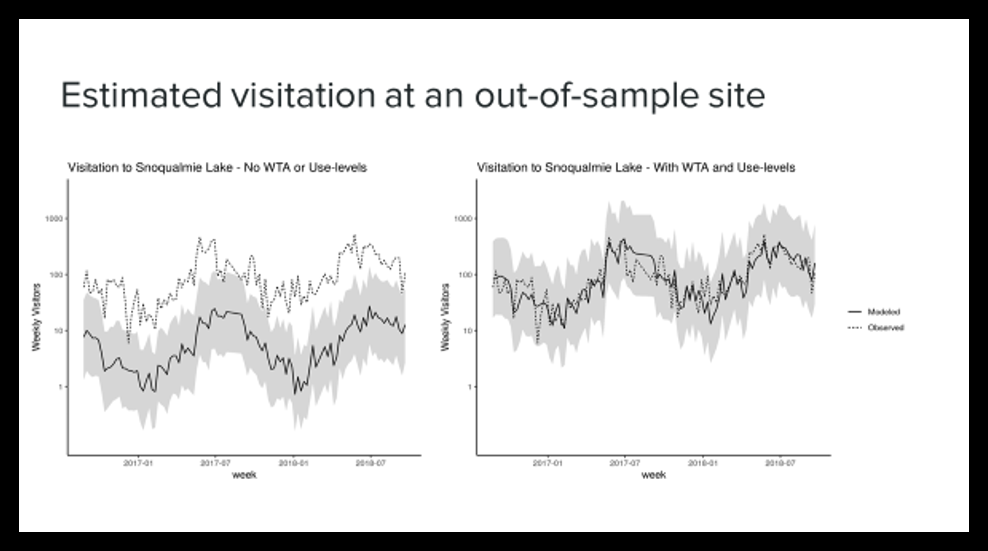Nowcasting with SAS Visual Forecasting Using Real Time Exogenous Variables

For best results, nowcasting with real time exogenous variables, use exogenous (input) variables that are highly correlated with your dependent variable. You will need to be able to obtain current or recent values for the input variables, obtain quality forecasts of the input variables, and collect the input variable data in real time.

Let’s follow Tammy Jackson’s example and see how we can accomplish this!

Tammy Jackson’s Beer Sales ExamplesTammy began with beer sales data from DurstExpress in Germany (Pan, W.H. 2022). She acquired daily data for 2019.

SECTION 1: USING FORECASTED AIR TEMPERATURE VERSUS ACTUAL AIR TEMPERATURE IN THE FORECAST PERIOD

Three exogenous factors are included in the data:

• hours of daylight
• public holiday (binomial variable where 0 = no or 1 = yes)
• daily mean air temperature in °C

In initially exploring the data of beer sales in Germany, we see that hours of daylight and mean air temperature are highly correlated with the number of crates of beer sold.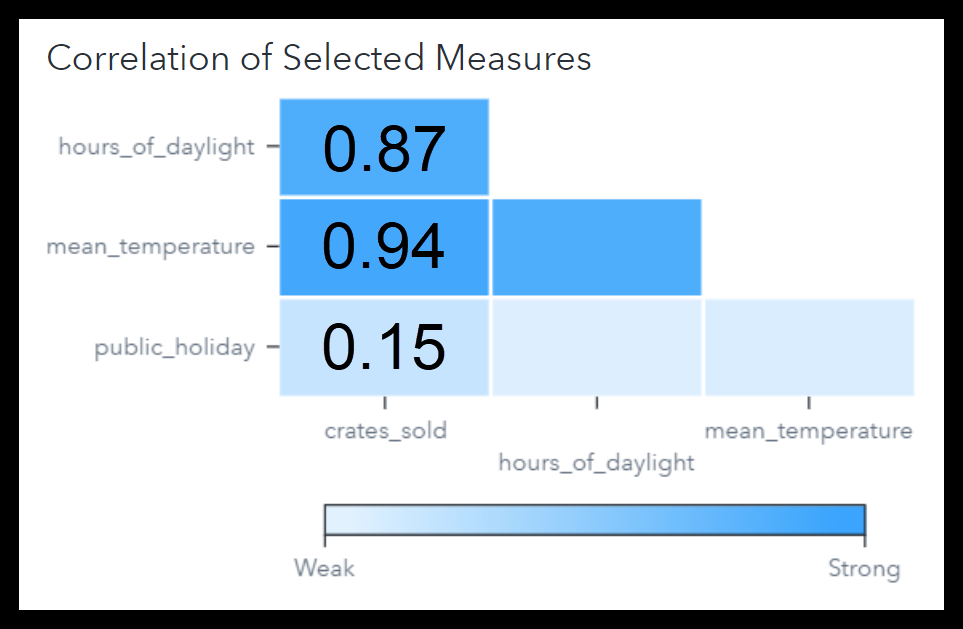These data are shown graphed below, where the:

• Blue circles are the actual data (crates of beer sold each date)
• Smooth black sinusoidal curve is the hours of daylight
• Jagged red line is the mean temperature
• Blue line at the bottom with nine peaks indicates public holidays

Note that Tammy scaled these to view them together.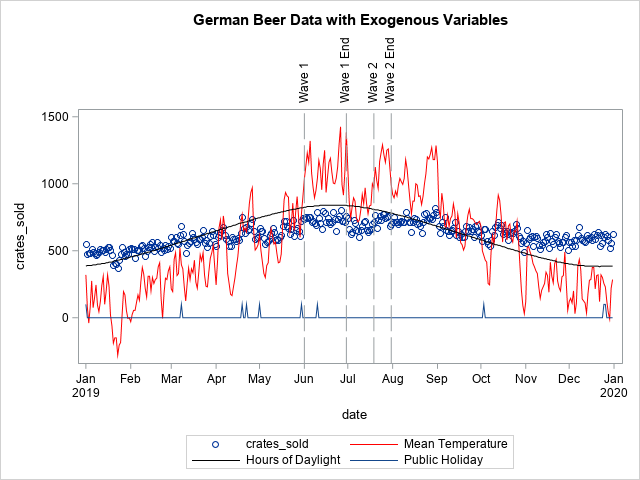By visually looking at the data we can zoom in on Wave One and Wave Two. Let’s fit a model to the German Beer Data and try several model families:

• ARIMA with regression variables
• Exponential smoothing
• Unobserved components with regression variables

And let’s see if the exogenous variables are significant.

• hours of daylight
• public holiday
• daily mean air temperature

We will use PROC TSMODEL. We will use rc = dataFrame.AddX() statements to add the exogenous variables and define how to treat them. See the code below:

``````proc tsmodel data = sascas1.germanbeerdatafull
LOGCONTROL= (ERROR = KEEP WARNING = KEEP NOTE=KEEP none=keep)
puttolog=yes
outlog=sascas1.outlog
outobj = (
outEST = sascas1.OUTEST_ind (replace = YES)
outfor = sascas1.outfor (replace = YES)
outstat = sascas1.outstat (replace = YES)
outsel=sascas1.outselect (replace = YES)
)
;
id date INTERVAL = day setmissing = MISSING trimid=right ;
var crates_sold hours_of_daylight public_holiday mean_temperature ;

require atsm; /* Use the automatic time series modeling (ATSM) package */
print outlog;

submit;

declare object dataFrame(tsdf);
declare object diagSpec(diagspec);
declare object diagnose(diagnose);
declare object inselect(selspec);
declare object forecast(foreng);

rc = dataFrame.Initialize();

/* Add Variable to be FORECAST */

/* Add INDEPENDENT exogenous variables to model */
/* All variables added will be considered as input by models that support independent variables */
/* You can add and remove the x variables just by commenting out the AddX method statements */
rc = dataFrame.AddX(hours_of_daylight, 'required', 'NO', 'EXTEND','stochastic');
rc = dataFrame.AddX(public_holiday, 'required', 'NO', 'EXTEND','stochastic');
rc = dataFrame.AddX(mean_temperature, 'required', 'NO', 'EXTEND','stochastic');
/* OPEN THE DIAGNOSE SPEC */
/* Add some ARIMA model info to consider ARIMA family of models */
rc = diagSpec.open(); if rc < 0 then do; stop; end;
rc = diagSpec.setTransform('TRANSFORM', 'NONE');
rc = diagSpec.setARIMAX('CRITERION', 'AIC');
rc = diagSpec.setARIMAX('IDENTIFY', 'ARIMA');
/* Add some ESM model info to consider ESM family of models */
rc = diagSpec.setESM('method', 'bestn'); if rc < 0 then do; stop; end;
/* Add some UCM model info to consider UCM family of models */
rc = diagSpec.setUCM(); if rc < 0 then do; stop; end;
rc = diagSpec.close(); if rc < 0 then do; stop; end;

/* Initializing from the dataFrame bring in the input variables */
rc = diagnose.Initialize(dataFrame);if rc < 0 then do; stop; end;
/* setSpec brings in the model families */
rc = diagnose.setSpec(diagSpec); if rc < 0 then do; stop; end;
rc = diagnose.Run(); if rc < 0 then do; stop; end;
ndiag = diagnose.nmodels();

/* Define selSpec object*/
/* Create a selection list */
rc = inselect.Open(ndiag);
rc = inselect.close();
/* Define forecast object*/
rc = forecast.Initialize(dataFrame); if rc < 0 then do; stop; end;
rc = forecast.setOption('LEAD',7); if rc < 0 then do; stop; end;
rc = forecast.setOption('ALPHA', 0.05);
if rc < 0 then do; stop; end;
rc = forecast.setOption('CRITERION','AIC');
if rc < 0 then do; stop; end;
/* Add the selection list to the forecast engine */
rc = forecast.AddFrom(inselect); if rc < 0 then do; stop; end;
rc = forecast.run(); if rc < 0 then do; stop; end;

/* Collect results */
declare object outEst(outEst);
declare object outfor(outfor);
declare object outsel(outselect);
declare object outstat(outstat);

rc = outEst.collect(forecast);
rc = outfor.collect(forecast);
rc = outstat.collect(forecast);
rc = outsel.collect(forecast);

endsubmit;
run;
quit;``````

Results for the entire year are shown below for the model ARIMA (111)(101)7 NOINT. We see that the p-values for the public holiday and the mean temperature are very low indicating that these are significant exogenous factors. Therefore they are used in the model.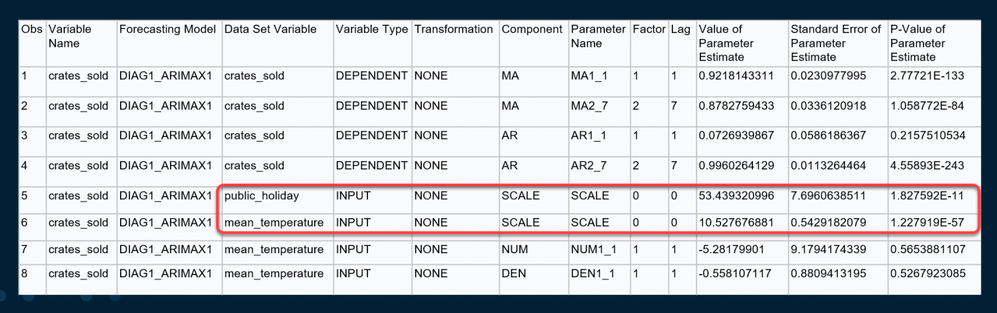The three models that were fitted are the ARIMAX, the ESM, and the UCM model. We can see in the screen shot below that the ARIMAX model did the best based on the Root Mean Square Error, the Mean Absolute Percent Error and the Akaike Information Criterion.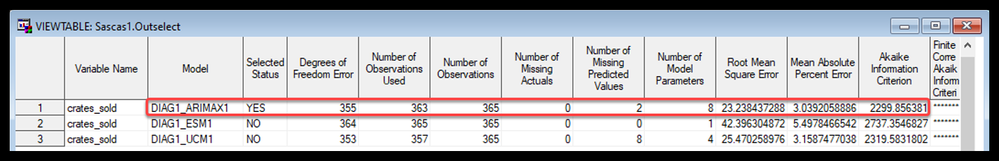Below we do see the forecast for the entire year and we see that the fit of the model over the year looks good. We do note annual seasonality, which we would capture if we had more years of data.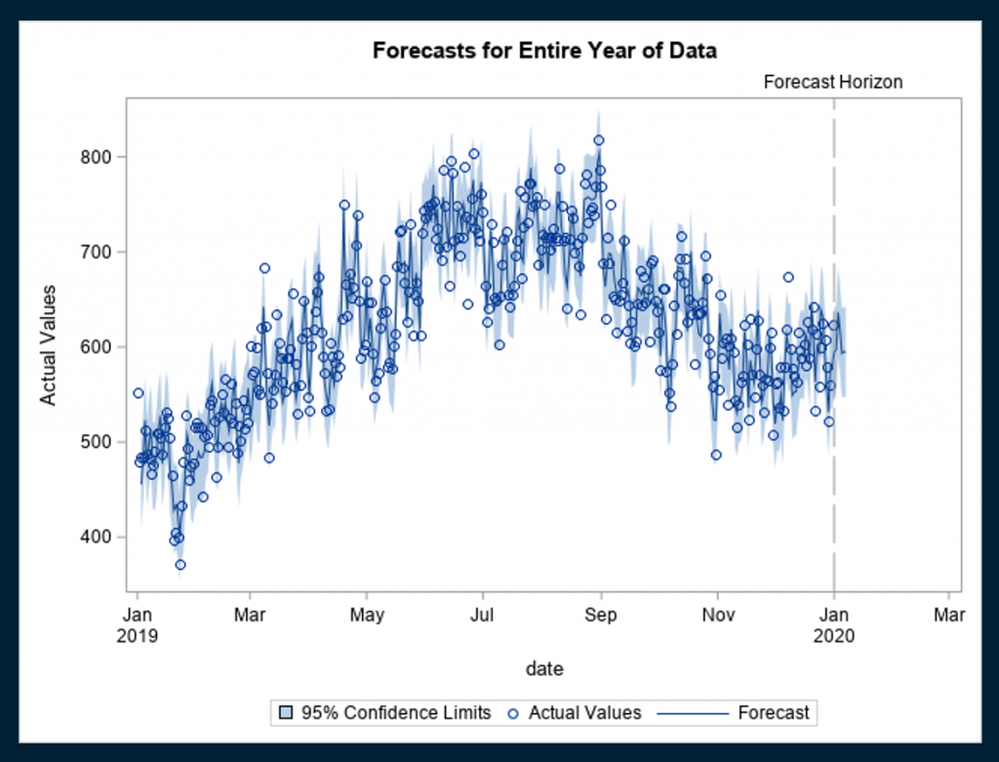Recall the two distinct waves in the data that Tammy identified visually.

Let’s forecast each of those waves. First, let use the entire year of data to identify a model. Then let’s use that model along with historical data (up to the point the wave starts) to forecast each wave. Mean temperature is an input and for one case we had missing values during forecast wave period. An exponential smoothing model will be used to forecast the temperature input. Let’s compare:

a) using an ESM forecast on the input (the stochastic forecast) versus
b) using the actual temperature data (as a proxy forecast)

In reality we would not have the actual data but would have pretty good National Weather Forecast at least one day in advance, so we are using the actual temperature data as a proxy for that.

First Wave Forecast

In the screen capture below, the red line is the forecast of number of crates of beer sold using the forecasted temperature input (the stochastic forecast). The blue line is the forecast of the number of crates of beer sold using the actual temperature data (as a proxy for the forecast of the independent variable temperature). As anticipated, we see that using the actual temperature data as an input improves our forecast.

We see this visually.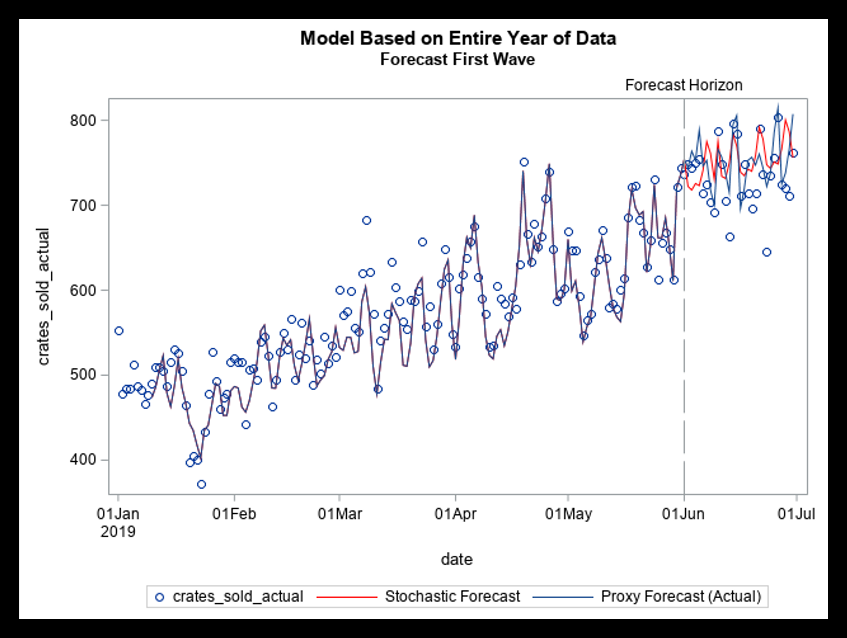And we confirm this by comparing the MAPE using the stochastic temperature forecast (perr_NF) versus using the actual temperature (perr_F), and we see that the forecast that used the actual temperature as in input during the forecast period is better.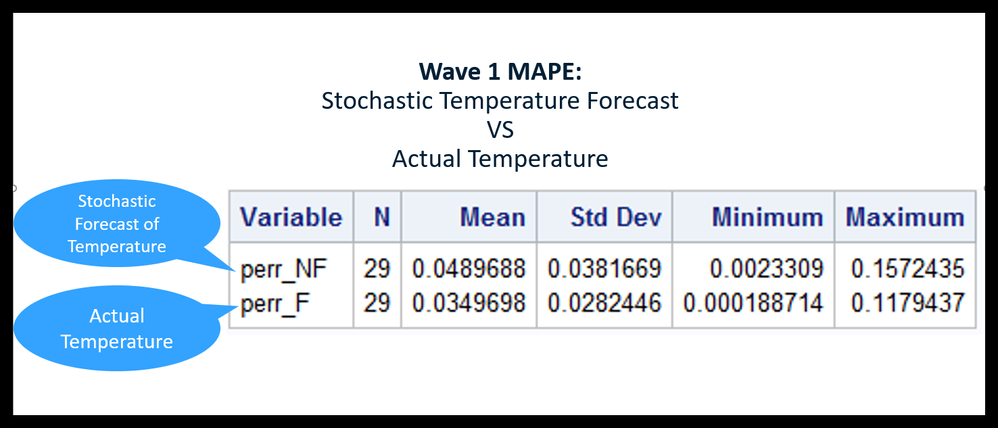Second Wave Forecast

We repeat this on the second wave of data. Again, we see that using the actual temperature data as an input improves our forecast.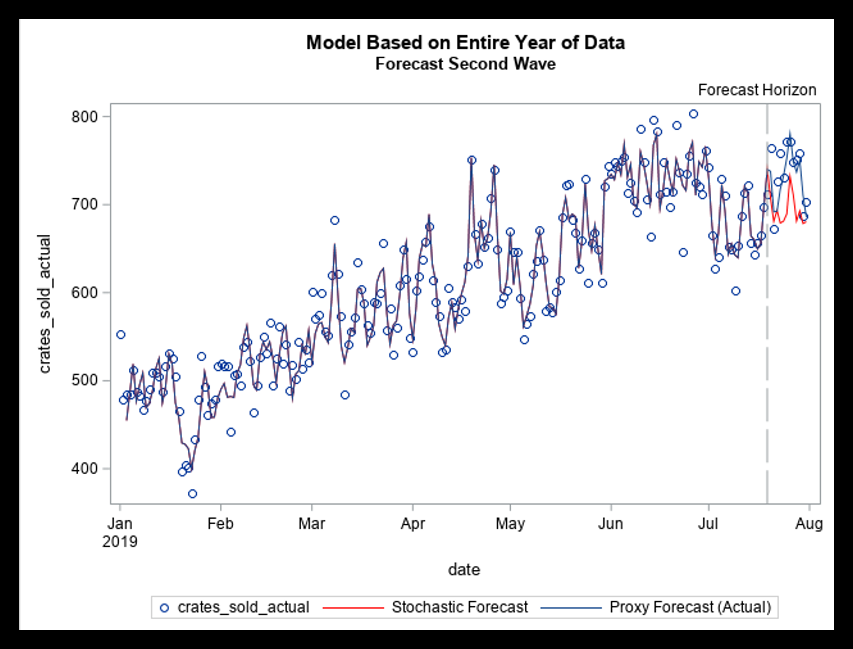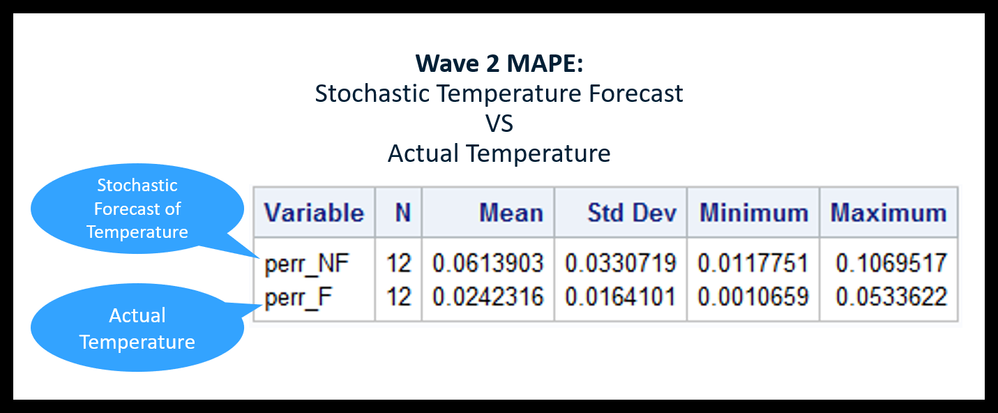SECTION 2: PULLING IN ONLINE DATA WITHIN PROC TSMODEL

In the above example it would have been useful to have recent high quality forecast of the temperature data to facilitate nowcasting.

Now that we are convinced using online real time data can improve our forecasts, how do we pull it in to SAS Visual Forecasting in real time and integrate the real time data into our forecast?

We can do it using SAS’s EXTLANG package for python developed by Javier Delgado and Thiago Quirino at SAS. Let’s illustrate this using historic weather data available from the US National Weather Service’s National Centers for Environmental Information (NCEI). The screen capture below shows the web interface for that NWS data, however we will be pulling this in using python and the EXTLANG package.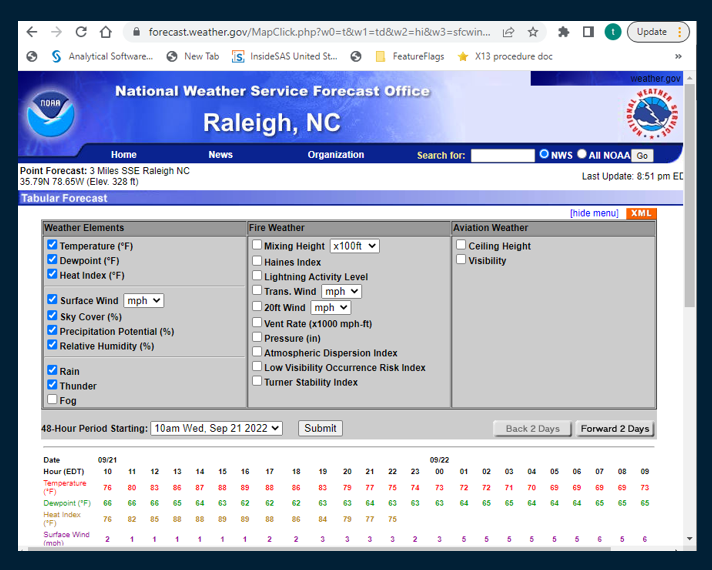There are a few pre-steps:

1. Download pyncei 1.0 and install in Python. You can get a token for access here .

2. A python package named pytrends works with Google trends to download weather data via the internet. We use it to download data from the US National Oceanic and Atmospheric Administration (NOAA). We must install pytrends prior to creating our SAS program, because we cannot have a pip install statement within PROC TSMODEL. See below using a Jupyter notebook with the statement pip install pytrends.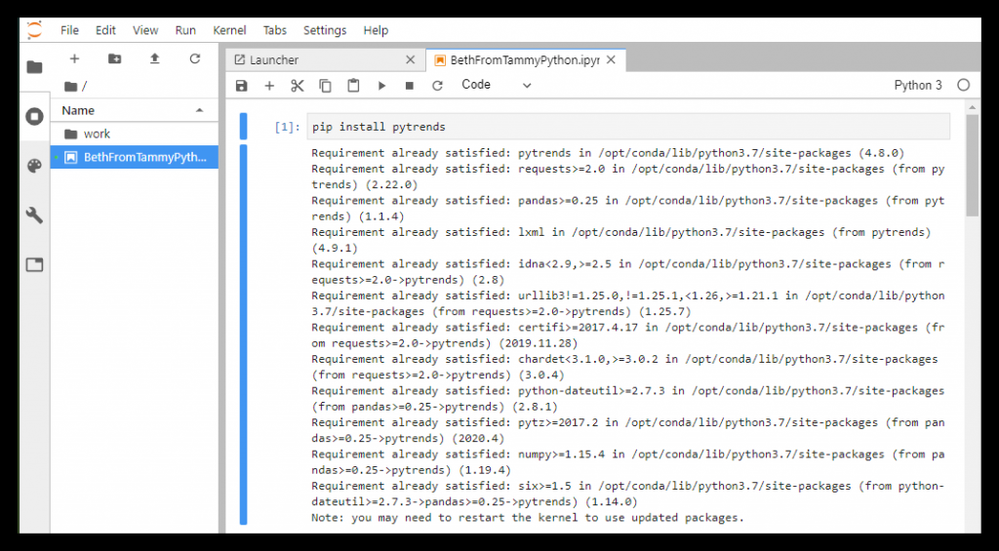3. Ensure that your administrator has enabled the SAS EXTLANG package in your environment. See more details at the end of this blog and in the documentation for more information.

So now we can use python WITHIN PROC TSMODEL to pull in internet data! The following code illustrates this by pulling in US National Oceanic and Administration (NOAA) data using a package from NOAA. In this example we pull in the data as a csv and then import it. But we could also pull in the data using arrays, as we will demonstrate later on in the Search Term example.

``````proc tsmodel data = sascas1.obs1
outobj = (
pylog=sascas1.pylog (replace = YES)
)
LOGCONTROL= (ERROR = KEEP WARNING = KEEP NOTE=KEEP none=keep)
puttolog=yes
outlog=sascas1.outlog
errorstop = YES lead = 11
id date INTERVAL = day ;
var crates_sold;

/* Use the External Language (EXTLANG) package as well as the ATSM package */
require atsm extlang;
print outlog;
submit;
declare object py(PYTHON3);
declare object pylog(OUTEXTLOG);
rc = py.Initialize();
rc = py.PushCodeLine('from noaa_sdk import NOAA');
rc = py.PushCodeLine('from numpy');
rc = py.PushCodeLine('from array import *');
rc = py.PushCodeLine('import pandas as pd');
rc = py.PushCodeLine('import numpy as np');
rc = py.PushCodeLine("keywords = ['startTime', 'temperature']");
rc = py.PushCodeLine("row=[]");
rc = py.PushCodeLine("n = NOAA()");
rc = py.PushCodeLine("res = n.get_forecasts('27615', 'US')");
rc = py.PushCodeLine("a = [ ['startTime', 'temperature'] ]");
rc = py.PushCodeLine("j=1");
rc = py.PushCodeLine("for i in res:");
rc = py.PushCodeLine(" row = [i[keywords],i[keywords]]");
rc = py.PushCodeLine(" a.insert(j, row)");
rc = py.PushCodeLine(" j=j+1");
rc = py.PushCodeLine("pd.DataFrame(a).to_csv("V:\<path>\temperaturefcsts.csv",index=False)");

/* Run */
rc = py.Run();
rc = pylog.Collect(py,'EXECUTION');

ENDSUBMIT;
RUN;

title "Import Hourly Temperature Forecasts for Zipcode 27615";
proc import out=
datasets.nws_temperature_fcst(rename=(VAR1=timeid VAR2=temperature_fcst)) REPLACE
datafile="V:\presentations\FFC2022\tempfcsts.csv"
DBMS="csv";
getnames=no;
datarow=3;
run;
data datasets.nws_temperature_fcst;
set datasets.nws_temperature_fcst;
format timeid DATETIME.;
run;

proc print data=datasets.nws_temperature_fcst;
run;``````

And we have imported hourly observations of NOAA temperature forecast data.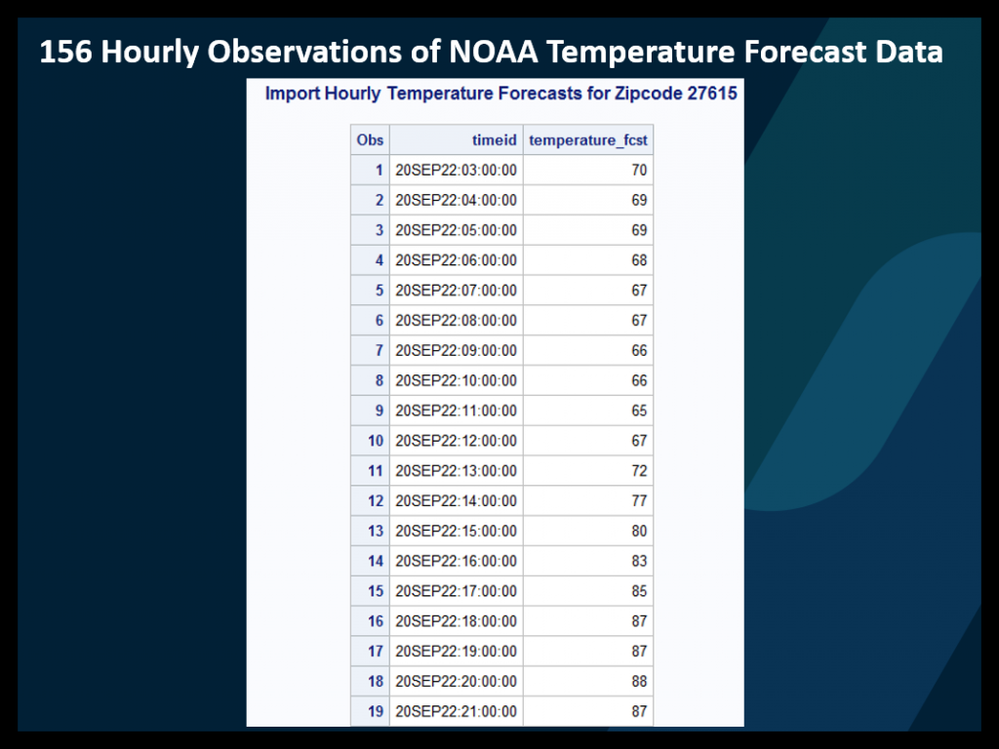If we want to accumulate those data to, for example, daily max, we could easily also do that in PROC TSMODEL.

``````/* Accumuate hourly forecasts to daily (max) temperature */
proc tsmodel data = sascas1.nws_temperature_fcst
LOGCONTROL= (ERROR = KEEP WARNING = KEEP NOTE=KEEP none=keep)
puttolog=yes
outlog=sascas1.outlog
outarray = sascas1.dailyTempFcst
errorstop = YES
;
id timeid INTERVAL = DTDAY setmissing = MISSING trimid=right ;
var temperature_fcst / acc=max;
require atsm;
print outlog;
submit;
declare object dataFrame(tsdf);
endsubmit;
run;
quit;``````

With results such as those shown below.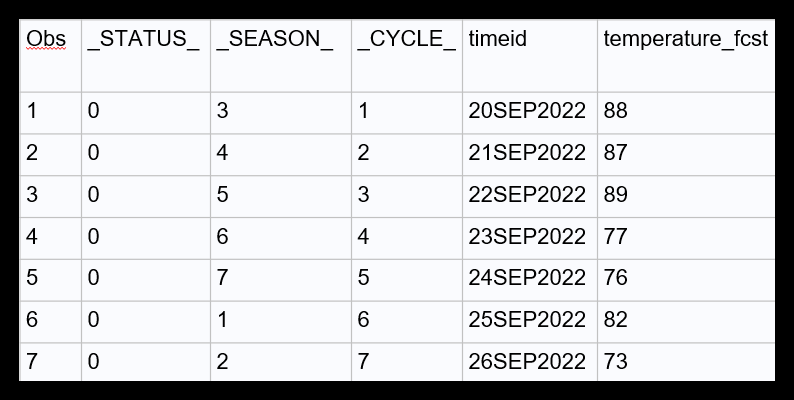SECTION 3: USING GOOGLE SEARCH TERM RECORDS AS EXOGENOUS INPUTS TO FORECAST BEER SALES WITHIN PROC TSMODEL

We will conduct three separate forecasts (nowcasts) within PROC TSMODEL. Once they are run we will compare the accuracies. In each forecast we will use different inputs to determine the amount of beer sold. For inputs we will use:

1. For forecast 1: Default forecast of mean temperature as inputs in the forecast period plus the search term magnitude also as inputs

2. For forecast 2: Actual temperatures as inputs in the forecast period plus the search term magnitude also as inputs

3. For forecast 3: Search term magnitude are the only inputs in the forecast period Let’s describe each of these in a little more detail.

The Google Trends Search Term data are based on the daily number of searches for each term. We see below data for daily searches for:

• “weather” (blue)
• “beer” (orange)
• “temperature (yellow)
• “hot” (green)

Rather than being represented as the actual number of searches, the Google Trends normalizes the data to a scale of 0 to 100 with 100 being the maximum number of daily searches for an individual term in the range of the data. So for example, we see in the Google trends data graph below that the highest number of daily searches was for weather and it occured in July. This date receives a value of 100 for weather searches and all other data are relative to that range maximum.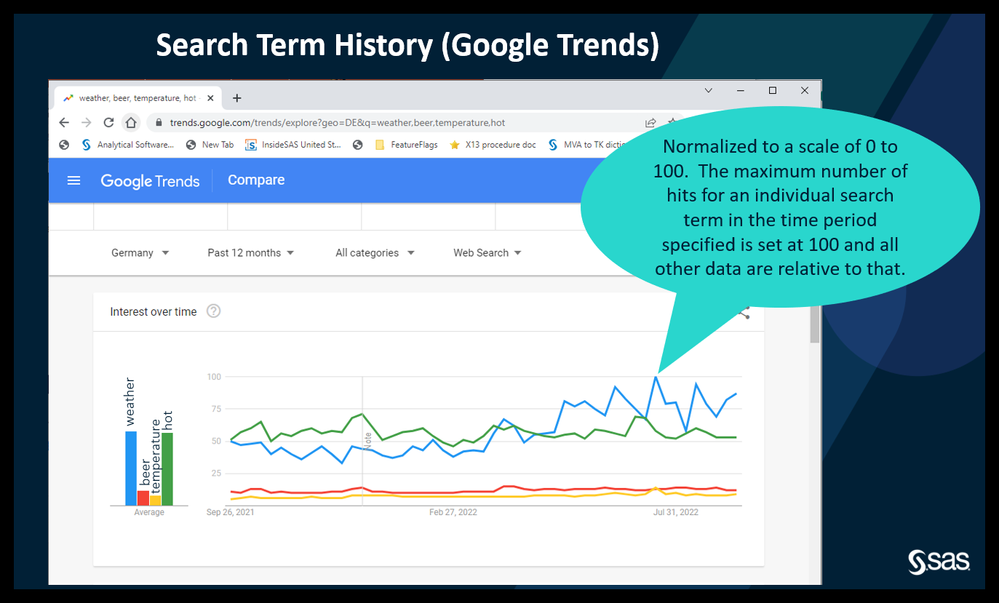1) DEFAULT FORECAST OF MEAN TEMPERATURE is used as an input for the forecast period in addition to the search term magnitudes. The default forecast (mean_temperature_NF) uses an ESM model to forecast the mean temperature during the forecast period. We also include search term data. The errors for this model are perr_NF. The results are collected as outfor in the code provided. Inputs available to Forecast 1 are:

• hours_of_daylight
• public_holiday
• mean_temperature_NF (modeled with ESM)
• search term data:
• BEER
• HEAT
• TEMPERATURE
• WEATHER

2) ACTUAL TEMPERATURE VALUES are used as the inputs for the forecast period in addition to the search term magnitudes. The errors for this are perr_F. The results are collected as outfor2 in the code provided. (Note: In real life you wouldn’t be able to use actual values for the current day, but you could use actual values up to the current day and a good weather forecast from the NWS for the current day.) Inputs available to Forecast 2 are:

• hours_of_daylight
• public_holiday
• mean_temperature (actual temperature values)
• search term data:
• BEER
• HEAT
• TEMPERATURE
• WEATHER

3) INPUTS ARE SEARCH TERM DATA ONLY In this case the forecast with mean_temperature is removed entirely, and ONLY the search term data for beer, heat, temperature, weather (normalized distributions) are used as inputs. The errors for this are perr_ST (where ST stands for search term.) The results are collected as outfor3. Inputs available to Forecast 3 are:

• public_holiday
• search term data:
• BEER
• HEAT
• TEMPERATURE
• WEATHER
• mean_temperature not available to the forecast model
• hours_of_daylight not available to the forecast model

When you get down to the results, you will be amazed to see that just using the search terms (forecast 3) gives a forecast just as good as when we use the ESM to forecast the mean temperature (forecast 1); in fact, it’s slightly better!

Keep in mind that the quality of the independent variables in the data makes a difference in the results during the forecast period.

Let’s get started: In your environment you will need to open a CAS session, identify your port, username, password, et cetera. I have not included code for that. If you need help with that starting code see the documentation.

Once we have opened a CAS session, we need libname statements to access and write data tables.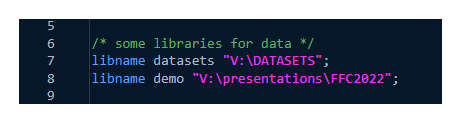We use a DATA STEP to pull in the time period we are interested in. Note that we must ensure that the input data set is the size of the desired output data set. It’s best if your input data set covers the same time period as the real time data that we pull in using python.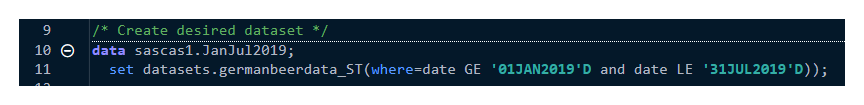Within the DATA STEP, let’s create time periods of interest (wave1 and wave2).We start our PROC TSMODEL and set up an outarray for all of our variables as shown in the screen capture below. We also create a pylog so that we can access log information from our python program after it runs. And we create some forecasting objects, typical of any forecasting project:

• parameter estimates
• outest
• outest2
• outest3
• forecasted values
• outfor
• outfor2
• outfor3
• model statistics
• outstat
• outstat2
• outstat3
• selection statistics
• outselect
• outselect2
• outselect3

Recall that we must ensure that the input data set is the size of the desired output data set. Below see that lead = 0 so that the size of the output data set doesn’t grow and therefore is the same as the size of the input data set.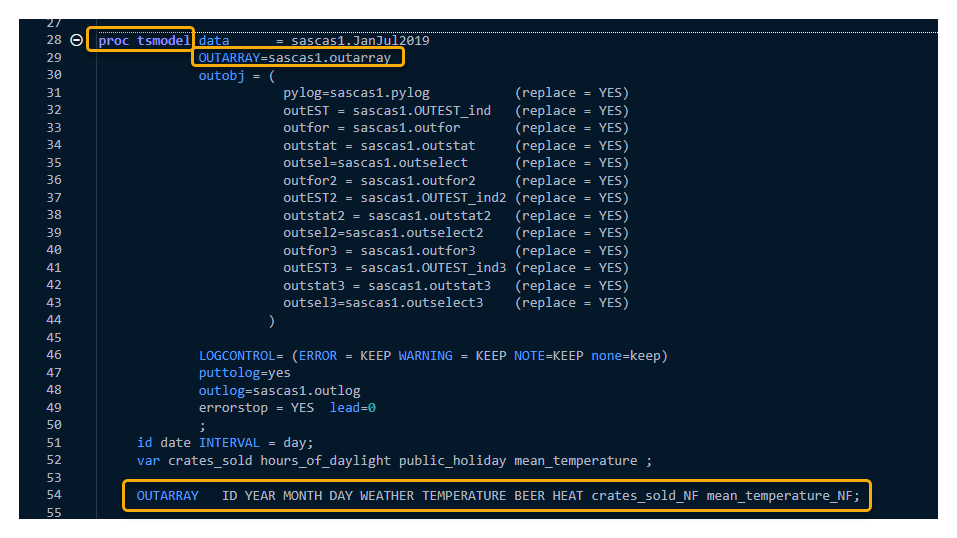As you see in the OUTARRAY statement above, our variables are:

• ID
• YEAR
• MONTH
• DAY
• WEATHER
• TEMPERATURE
• BEER
• HEAT
• crates_sold_NF
• mean_temperature_NF

During the forecast period actual values were removed and replaced with missing values for crates_sold_NF and mean_temperature_NF.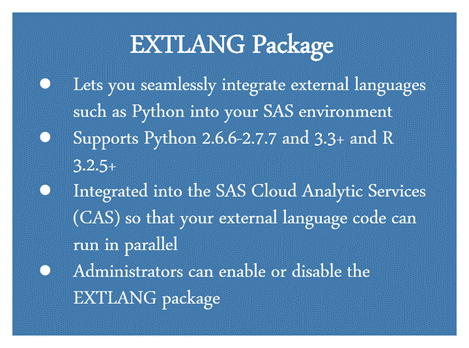Now, we need to require two packages, as shown in the screen shot below:

• ATSM – automatic time series modeling package
• EXTLANG – external languages package, which will allow us to use python programming language within our PROC TSMODEL code!

For more information on the EXTLANG package see the section on this at the end of this blog and see the documentation here and here.

With the EXTLANG package, we are now free to submit our user-defined code (both SAS code and Python code!) between the submit and endsubmit statements as shown in the screen capture below.

The first thing we do inside of our submit and endsubmit statements is to declare our python object and our python log, as shown in the screen capture above:

declare object py(PYTHON3);
declare object pylog(OUTEXTLOG);

This ensures that we can see a log in case of errors. We use return code statements to run the Python lines of code that we need. First let’s initialize python. Then we add the variables we need (to make them available to our python code), such as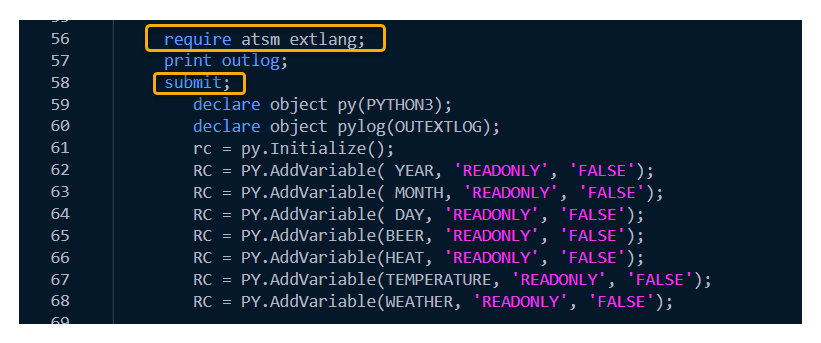Now we can submit the python code lines using PushCodeLine statements as shown in the screen capture below. We start by pulling in the python packages we need. Remember that we need to import the pytrends API so that we can pull in the NOAA data from the internet in real time.

Note that the python code is inside the quotes. If you have quotes within quotes, you need to make sure that either:

the exterior quotes are double quotes and the interior quotes are single quotes, or

the exterior quotes are single quotes and the interior quotes are double quotes.

For example:

rc = py.PushCodeLine(“pytrends = TrendReq(h1=’en-US’, tz=0, retries=10)”);

In the code snippet below we see the line where this is illustrated: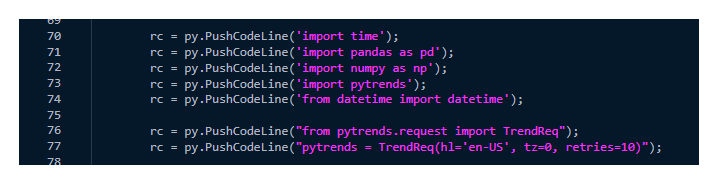We continue to use rc = py.PushCodeLine to select and sort keywords, select the geography (DE for Deutschland, which is Germany). We also set up a wait of 6 seconds.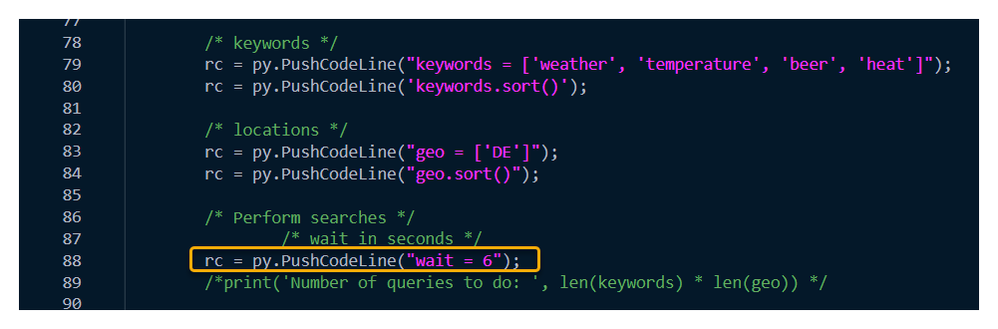In the screen capture below we prepare the containers and start loops to retrieve data using pytrends. We set up the data dictionary with the python code trends = dict.fromkeys(geo). We set up an empty error list with the code errors_list = [] which will populate if there are errors. We loop over the geographies and the keywords. Notice that we also include the python code time.sleep(wait) (set at 6 seconds in the screen capture above) to help avoid timeout errors.We will initialize the arrays to receive the data. Recall that we must ensure that the input data set is the size of the desired output data set. See below we use (212) to ensure that the arrays are the same size as the input and output data sets.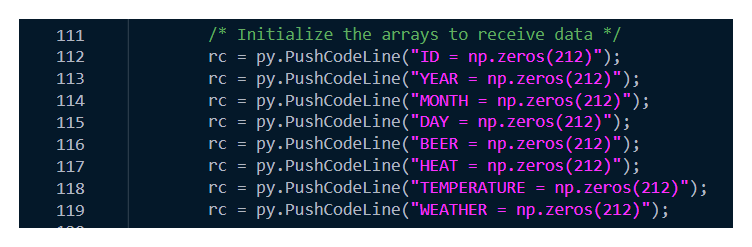Next we transfer the pytrends search term data to local arrays.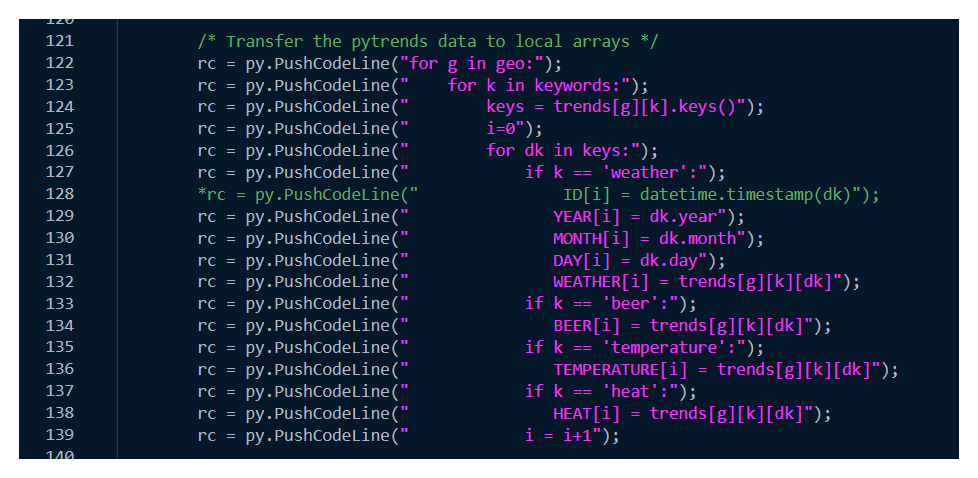And we run the python program and collect the python log information.Now we create the date time stamp for the imported data to ensure that the internet data that we have pulled in using pytrends are properly aligned with the time series data we are forecasting (beer sales data).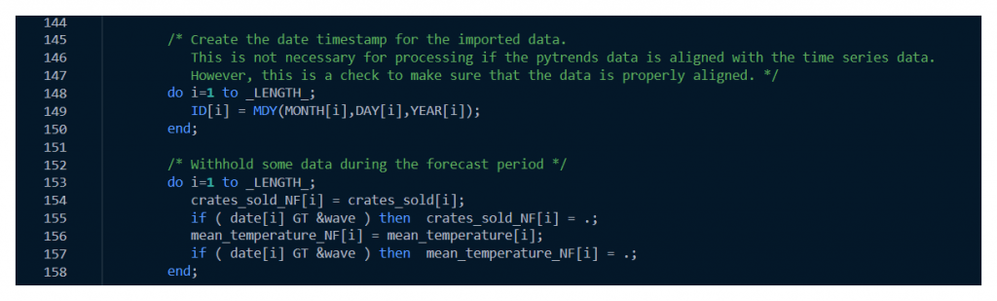Example output for the first four observations of the search term data is shown below.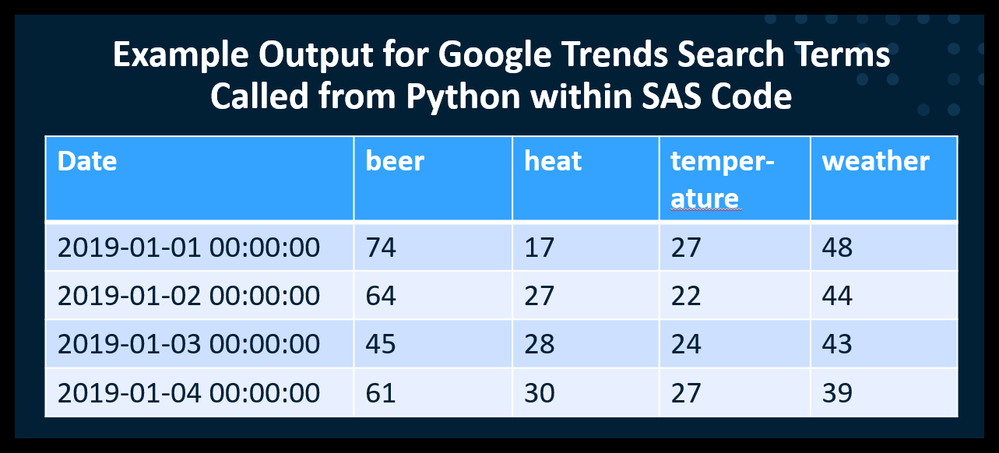Running the forecasts:

As in any PROC TS MODEL code we declare our objects including the FORENG object for our first forecast.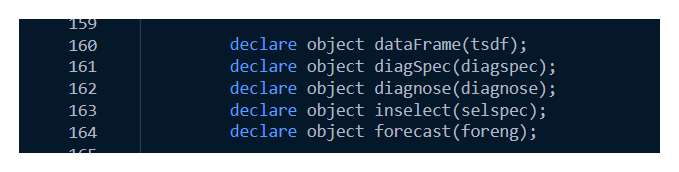We initialize our dataFrame and add our forecast variable, which is the number of crates sold crates_sold_NF. And here we extend using a stochastic model (an exponential smoothing model).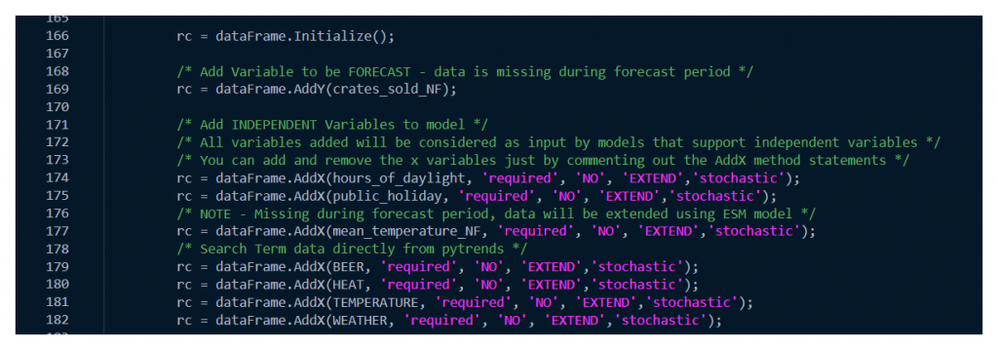We add some ARIMA model information including that we will use the Akaike Information Criterion as the model selection criterion.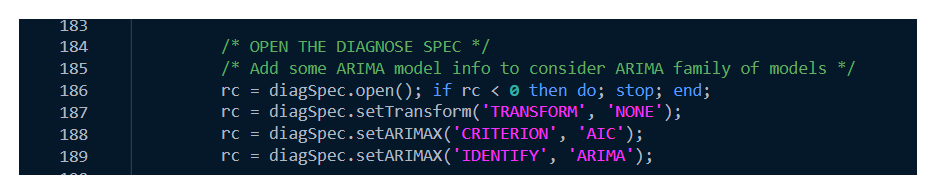We also add ESM and UCM models for consideration.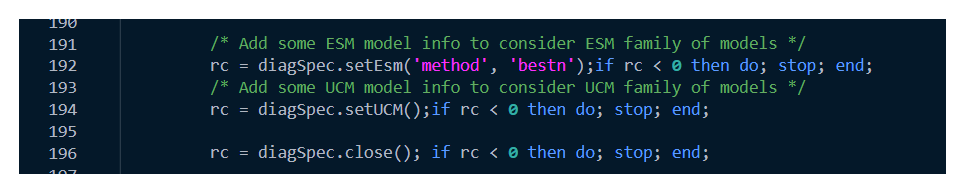Initializing from the dataFrame brings in the input variables.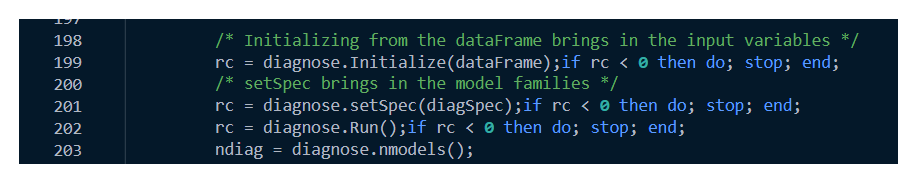Then we create a selection list.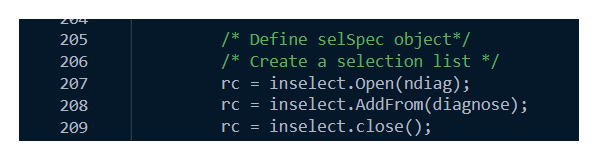We define our forecast object, set lead time using a macro variable, set alpha to 0.05, and set the selection criterion to Akaike Information Criterion. We will also add the selection list to the forecast engine.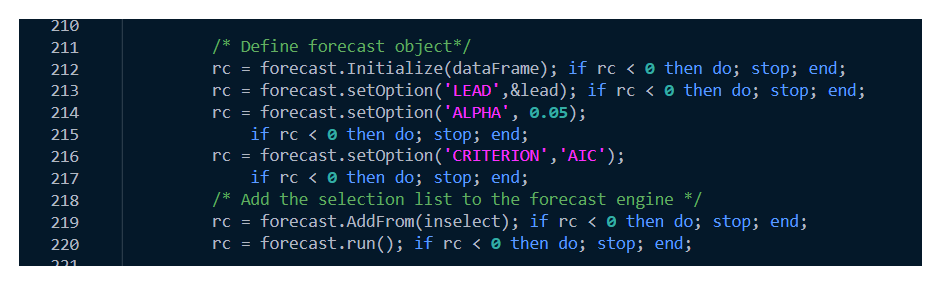And, as with all PROC TSMODEL code, we collect our results. Here ends the first forecast.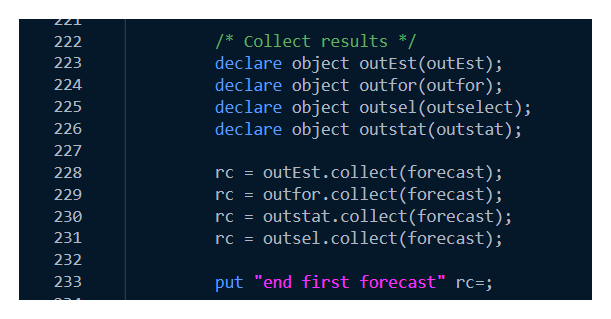We repeat similar code for forecast 2.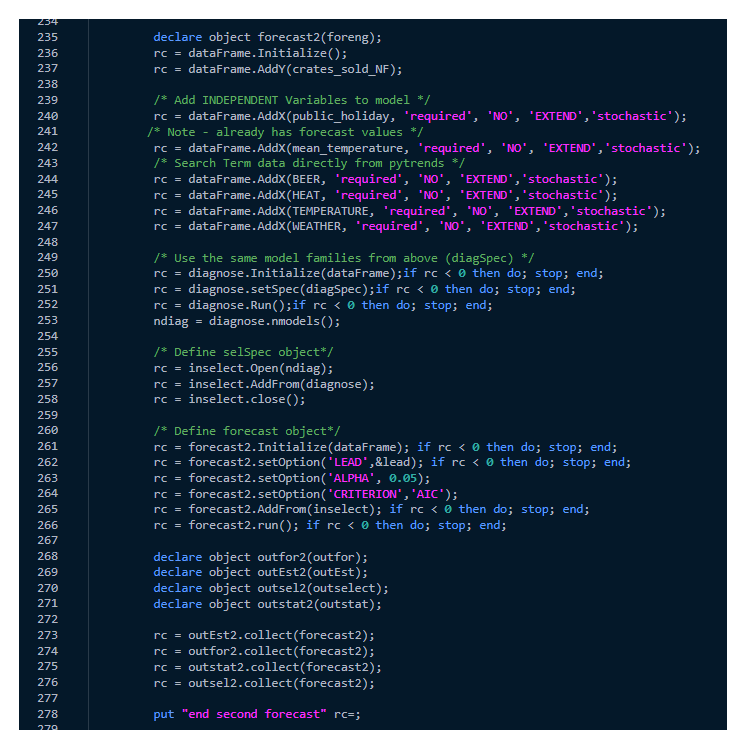Repeat for forecast 3.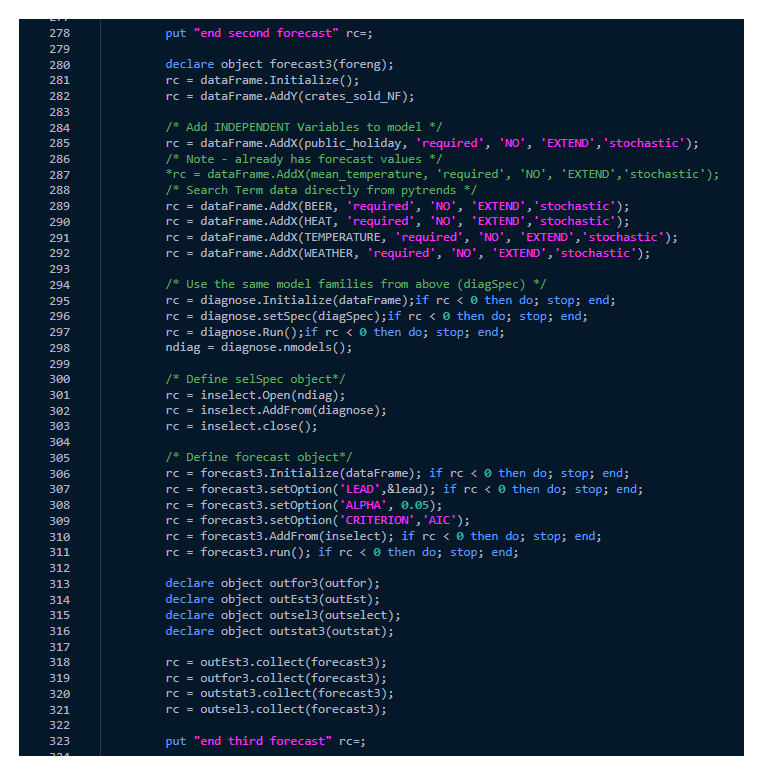And finally our endsubmit statement, which ends all of our user-defined code.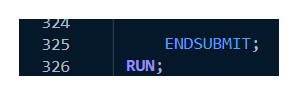We use some DATA STEP code to create three data sets with the information from our three forecasts:
• forecast1
• forecast2 (forecast with actual inputs from the internet)
• forecast3 (forecast with stochastically forecasted inputs)

We can then print and compare the results.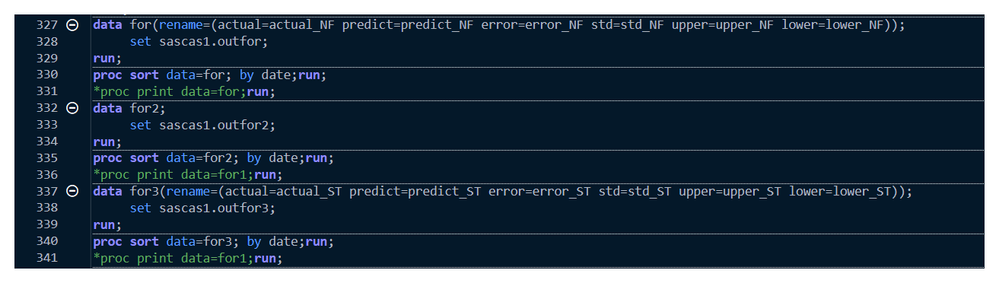Results

We merge our three forecast tables and create variables for us to look at:
• perr_NF – the percent error for the model built with the ESM-modeled mean_temperature as an input
• perr_F – the percent error for the proxy model (forecast with actual mean_temperature data from the internet)
• perr_ST – the percent error for the model that uses only the search term data as inputs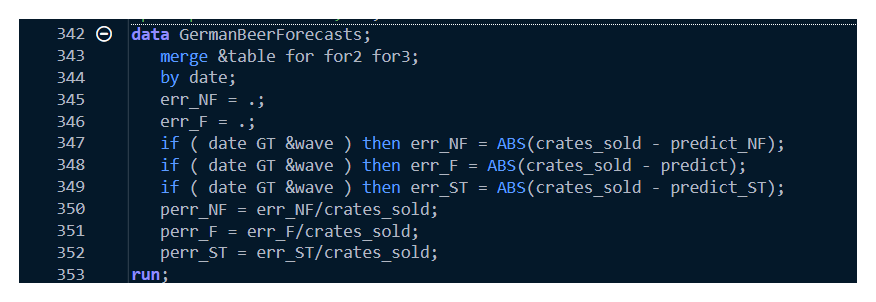Plot out stochastic and proxy forecast results.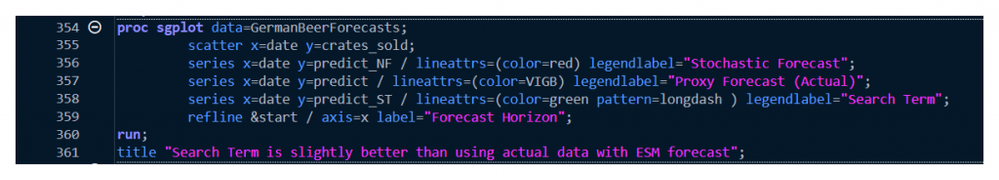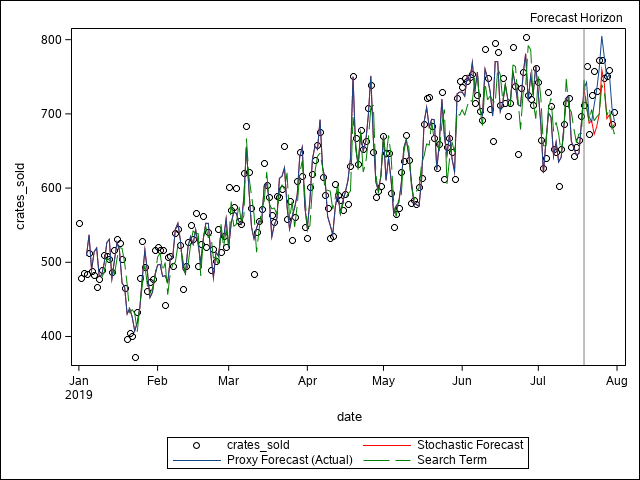Compare the means of the errors.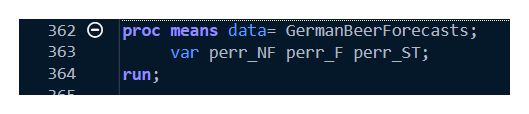Evaluating the errors using PROC MEANS, we see that:
• perr_NF is the worst. Recall that perr_NF is the percent error for the model built with the ESM-modeled mean_temperature as an input • perr_F is the best. Recall that perr_F is the percent error for the proxy model (forecast with actual mean_temperature data from the internet)
• perr_ST is slightly better than perr_NF! Recall that perr_ST is the percent error for the model that uses

ONLY the search term data as inputs. We find that even though the search terms didn’t fit as well when modeled together with the other inputs, they were slightly better predictors when used alone.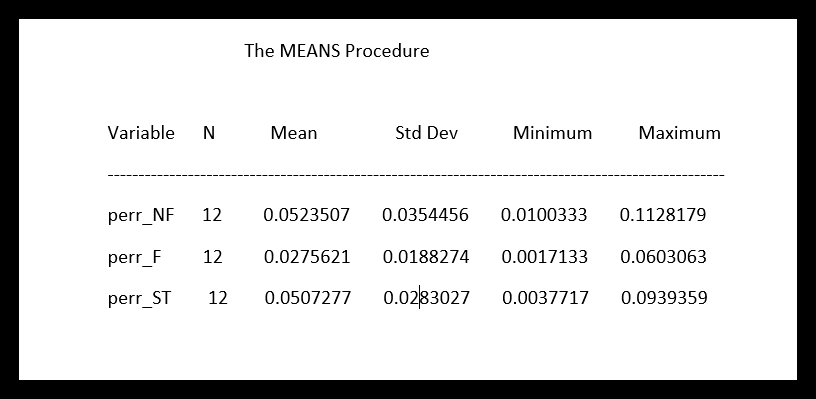Conclusions

• Data available in real time can be successfully incorporated into models to produce quality forecasts.
• The quality of the forecast of the exogenous variables can have a major impact on the quality of the forecasts of the dependent variable.
• Free data are available, such as
• Historical weather – US National Centers for Environmental Information (NCEI)
• Current weather forecasts – US National Weather Service (NWS)
• Internet search term data – Google Trends
• All of the above data can be accessed via Python within PROC TSMODEL
• Integrating Python code into the forecasting process is necessary to produce a quality forecast in real time

Summary

We see that we were able to get good nowcasting results by incorporating data from the internet. We did this directly in SAS Visual Forecasting using PROC TSMODEL and the EXTLANG package that allows us to include python code in our user-defined code. Again, let me reiterate. We are able to bring in internet data and use it directly in SAS Visual Forecasting within PROC TSMODEL. This is a game changer.

A huge thank you to Tammy Jackson, SAS Principal Research Statistician Developer, for demonstrating how this is possible. Thank you also to Javier Delgado and Thiago Quirino for developing the EXTLANG package that makes this possible. Full Code to run the 3 forecasts :

``````/*********************************** Beth Open CAS Code **************************************/
/* REPLACE THIS WITH YOUR OWN STARTING CODE */
cas BethSession sessopts=(caslib=casuser timeout=1800 locale="en_US");
*libname ANALYTIC cas caslib = “ANALYTIC”;
*libname VF cas caslib="VF";
libname CASUSER cas caslib="CASUSER";
*cas Sessionxxx terminate;

Data PUBLIC.germanbeerfull (drop = ST_weather_O ST_temperature_O ST_beer ST_temperature ST_weather);
set PUBLIC.GERMANBEERDATA_ST;
proc print;
run;
/*********************************************************************************************/

/* German Beer Data January 1, 2019 to July 31, 2019 */
data PUBLIC.JanJul2019;
set PUBLIC.germanbeerdata_ST(where=(date GE '01JAN2019'D and date LE '31JUL2019'D));
run;
/* Some time periods of interest */
%let wave1 = '01JUN2019'D;
%let wave1end = '30JUN2019'D;
%let wave2 = '19JUL2019'D;
%let wave2end = '31JUL2019'D;

%let table = PUBLIC.JanJul2019;
%let start = &wave2;
%let wave = &wave2;

/*********************************************************************************************/
/* PROC TSMODEL STARTS HERE */
/*********************************************************************************************/

proc tsmodel data = PUBLIC.JanJul2019
OUTARRAY = PUBLIC.outarray
outobj = (
pylog = PUBLIC.pylog (replace = YES)
outEST = PUBLIC.OUTEST_ind (replace = YES)
outfor = PUBLIC.outfor (replace = YES)
outstat = PUBLIC.outstat (replace = YES)
outsel = PUBLIC.outselect (replace = YES)
outfor2 = PUBLIC.outfor2 (replace = YES)
outEST2 = PUBLIC.OUTEST_ind2 (replace = YES)
outstat2 = PUBLIC.outstat2 (replace = YES)
outsel2 = PUBLIC.outselect2 (replace = YES)
outfor3 = PUBLIC.outfor3 (replace = YES)
outEST3 = PUBLIC.OUTEST_ind3 (replace = YES)
outstat3 = PUBLIC.outstat3 (replace = YES)
outsel3 = PUBLIC.outselect3 (replace = YES)
)

LOGCONTROL= (ERROR = KEEP WARNING = KEEP NOTE=KEEP none=keep)
puttolog=yes
outlog=PUBLIC.outlog
;
id date INTERVAL = day;
var crates_sold hours_of_daylight public_holiday mean_temperature ;

OUTARRAY ID YEAR MONTH DAY WEATHER TEMPERATURE BEER HEAT crates_sold_NF mean_temperature_NF;

require atsm extlang;
print outlog;
submit;
declare object py(PYTHON3);
declare object pylog(OUTEXTLOG);
rc = py.Initialize();
/* This install needs to be done prior to running SAS code */
*rc = py.PushCodeLine('!pip install pytrends');

rc = py.PushCodeLine('import time');
rc = py.PushCodeLine('import pandas as pd');
rc = py.PushCodeLine('import numpy as np'); *BETH ADDED THIS LINE;
rc = py.PushCodeLine('import pytrends');
rc = py.PushCodeLine('from datetime import datetime');

rc = py.PushCodeLine("from pytrends.request import TrendReq");
rc = py.PushCodeLine("pytrends = TrendReq(hl='en-US', tz=0, retries=10)");

/* keywords */
rc = py.PushCodeLine("keywords = ['weather', 'temperature', 'beer', 'heat']");
/* Sort the list */
rc = py.PushCodeLine('keywords.sort()');

/* locations */
rc = py.PushCodeLine("geo = ['DE']");
rc = py.PushCodeLine("geo.sort()");

/* Perform searches */
/* wait in seconds */
rc = py.PushCodeLine("wait = 6");
/*print('Number of queries to do: ', len(keywords) * len(geo)) */

/*# Prepare containers */
rc = py.PushCodeLine("trends = dict.fromkeys(geo)");
rc = py.PushCodeLine("errors_list = []");
rc = py.PushCodeLine("cnt = 1");

/* Start loops to retrieve data from pytrends */
rc = py.PushCodeLine("for g in geo:");
rc = py.PushCodeLine(" trends[g] = {}");
rc = py.PushCodeLine(" for k in keywords:");
rc = py.PushCodeLine(" try:");
rc = py.PushCodeLine(" time.sleep(wait)");
rc = py.PushCodeLine(" pytrends.build_payload([k], timeframe='2019-01-01 2019-07-31', geo=g, gprop='')");
rc = py.PushCodeLine(" trends[g][k] = pytrends.interest_over_time()[k]");

rc = py.PushCodeLine(" except:");
/*print(cnt, ') Error: ', g, ' & ', k)*/
rc = py.PushCodeLine(" errors_list.append([g,k])");
rc = py.PushCodeLine(" cnt+=1");

/*print('\nDone -', len(errors_list), 'errors left')*/

/* Initialize the arrays to receive data */
rc = py.PushCodeLine("ID = np.zeros(212)"); *BETH ADDED THIS LINE;
rc = py.PushCodeLine("YEAR = np.zeros(212)");
rc = py.PushCodeLine("MONTH = np.zeros(212)");
rc = py.PushCodeLine("DAY = np.zeros(212)");
rc = py.PushCodeLine("BEER = np.zeros(212)");
rc = py.PushCodeLine("HEAT = np.zeros(212)");
rc = py.PushCodeLine("TEMPERATURE = np.zeros(212)");
rc = py.PushCodeLine("WEATHER = np.zeros(212)");

/* Transfer the pytrends data to local arrays */
rc = py.PushCodeLine("for g in geo:");
rc = py.PushCodeLine(" for k in keywords:");
rc = py.PushCodeLine(" keys = trends[g][k].keys()");
rc = py.PushCodeLine(" i=0");
rc = py.PushCodeLine(" for dk in keys:");
rc = py.PushCodeLine(" if k == 'weather':");
*rc = py.PushCodeLine(" ID[i] = datetime.timestamp(dk)");
rc = py.PushCodeLine(" YEAR[i] = dk.year");
rc = py.PushCodeLine(" MONTH[i] = dk.month");
rc = py.PushCodeLine(" DAY[i] = dk.day");
rc = py.PushCodeLine(" WEATHER[i] = trends[g][k][dk]");
rc = py.PushCodeLine(" if k == 'beer':");
rc = py.PushCodeLine(" BEER[i] = trends[g][k][dk]");
rc = py.PushCodeLine(" if k == 'temperature':");
rc = py.PushCodeLine(" TEMPERATURE[i] = trends[g][k][dk]");
rc = py.PushCodeLine(" if k == 'heat':");
rc = py.PushCodeLine(" HEAT[i] = trends[g][k][dk]");
rc = py.PushCodeLine(" i = i+1");

/* Run */
rc = py.Run();
rc = pylog.Collect(py,'EXECUTION');

/* Create the date timestamp for the imported data.
This is not necessary for processing if the pytrends data is aligned with the time series data.
However, this is a check to make sure that the data is properly aligned. */
do i=1 to _LENGTH_;
ID[i] = MDY(MONTH[i],DAY[i],YEAR[i]);
end;

/* Withhold some data during the forecast period */
do i=1 to _LENGTH_;
crates_sold_NF[i] = crates_sold[i];
if ( date[i] GT &wave ) then crates_sold_NF[i] = .;
mean_temperature_NF[i] = mean_temperature[i];
if ( date[i] GT &wave ) then mean_temperature_NF[i] = .;
end;

declare object dataFrame(tsdf);
declare object diagSpec(diagspec);
declare object diagnose(diagnose);
declare object inselect(selspec);

/**********************************************************************************************************/
/* CODE FOR FIRST FORECAST: Uses default forecast (ESM) of temperatures as inputs in the forecast period */
/**********************************************************************************************************/
declare object forecast(foreng);

rc = dataFrame.Initialize();

/* Add Variable to be FORECAST - data is missing during forecast period */

/* Add INDEPENDENT Variables to model */
/* All variables added will be considered as input by models that support independent variables */
/* You can add and remove the x variables just by commenting out the AddX method statements */
rc = dataFrame.AddX(hours_of_daylight, 'required', 'NO', 'EXTEND','stochastic');
rc = dataFrame.AddX(public_holiday, 'required', 'NO', 'EXTEND','stochastic');
/* NOTE - Missing during forecast period, data will be extended using ESM model */
rc = dataFrame.AddX(mean_temperature_NF, 'required', 'NO', 'EXTEND','stochastic');
/* Search Term data directly from pytrends */
rc = dataFrame.AddX(BEER, 'required', 'NO', 'EXTEND','stochastic');
rc = dataFrame.AddX(HEAT, 'required', 'NO', 'EXTEND','stochastic');
rc = dataFrame.AddX(TEMPERATURE, 'required', 'NO', 'EXTEND','stochastic');
rc = dataFrame.AddX(WEATHER, 'required', 'NO', 'EXTEND','stochastic');

/* OPEN THE DIAGNOSE SPEC */
/* Add some ARIMA model info to consider ARIMA family of models */
rc = diagSpec.open(); if rc < 0 then do; stop; end;
rc = diagSpec.setTransform('TRANSFORM', 'NONE');
rc = diagSpec.setARIMAX('CRITERION', 'AIC');
rc = diagSpec.setARIMAX('IDENTIFY', 'ARIMA');

/* Add some ESM model info to consider ESM family of models */
rc = diagSpec.setEsm('method', 'bestn');if rc < 0 then do; stop; end;
/* Add some UCM model info to consider UCM family of models */
rc = diagSpec.setUCM();if rc < 0 then do; stop; end;

rc = diagSpec.close(); if rc < 0 then do; stop; end;

/* Initializing from the dataFrame brings in the input variables */
rc = diagnose.Initialize(dataFrame);if rc < 0 then do; stop; end;
/* setSpec brings in the model families */
rc = diagnose.setSpec(diagSpec);if rc < 0 then do; stop; end;
rc = diagnose.Run();if rc < 0 then do; stop; end;
ndiag = diagnose.nmodels();

/* Define selSpec object*/
/* Create a selection list */
rc = inselect.Open(ndiag);
rc = inselect.close();

/* Define forecast object*/
rc = forecast.Initialize(dataFrame); if rc < 0 then do; stop; end;
rc = forecast.setOption('ALPHA', 0.05);
if rc < 0 then do; stop; end;
rc = forecast.setOption('CRITERION','AIC');
if rc < 0 then do; stop; end;
/* Add the selection list to the forecast engine */
rc = forecast.AddFrom(inselect); if rc < 0 then do; stop; end;
rc = forecast.run(); if rc < 0 then do; stop; end;

/* Collect results */
declare object outEst(outEst);
declare object outfor(outfor);
declare object outsel(outselect);
declare object outstat(outstat);

rc = outEst.collect(forecast);
rc = outfor.collect(forecast);
rc = outstat.collect(forecast);
rc = outsel.collect(forecast);

put "end first forecast" rc=;

/****************************************************************************************/
/* CODE FOR SECOND FORECAST: Uses actual temperatures as inputs in the forecast period */
/****************************************************************************************/

declare object forecast2(foreng);
rc = dataFrame.Initialize();

/* Add INDEPENDENT Variables to model */
rc = dataFrame.AddX(public_holiday, 'required', 'NO', 'EXTEND','stochastic');
/* Note - already has forecast values */
rc = dataFrame.AddX(mean_temperature, 'required', 'NO', 'EXTEND','stochastic');
/* Search Term data directly from pytrends */
rc = dataFrame.AddX(BEER, 'required', 'NO', 'EXTEND','stochastic');
rc = dataFrame.AddX(HEAT, 'required', 'NO', 'EXTEND','stochastic');
rc = dataFrame.AddX(TEMPERATURE, 'required', 'NO', 'EXTEND','stochastic');
rc = dataFrame.AddX(WEATHER, 'required', 'NO', 'EXTEND','stochastic');

/* Use the same model families from above (diagSpec) */
rc = diagnose.Initialize(dataFrame);if rc < 0 then do; stop; end;
rc = diagnose.setSpec(diagSpec);if rc < 0 then do; stop; end;
rc = diagnose.Run();if rc < 0 then do; stop; end;
ndiag = diagnose.nmodels();

/* Define selSpec object*/
rc = inselect.Open(ndiag);
rc = inselect.close();

/* Define forecast object*/
rc = forecast2.Initialize(dataFrame); if rc < 0 then do; stop; end;
rc = forecast2.setOption('ALPHA', 0.05);
rc = forecast2.setOption('CRITERION','AIC');
rc = forecast2.AddFrom(inselect); if rc < 0 then do; stop; end;
rc = forecast2.run(); if rc < 0 then do; stop; end;

declare object outfor2(outfor);
declare object outEst2(outEst);
declare object outsel2(outselect);
declare object outstat2(outstat);

rc = outEst2.collect(forecast2);
rc = outfor2.collect(forecast2);
rc = outstat2.collect(forecast2);
rc = outsel2.collect(forecast2);

put "end second forecast" rc=;

/*************************************************************************************************/
/* CODE FOR THIRD FORECAST: Uses search term data distributions as inputs in the forecast period */
/*************************************************************************************************/

declare object forecast3(foreng);
rc = dataFrame.Initialize();

/* Add INDEPENDENT Variables to model */
rc = dataFrame.AddX(public_holiday, 'required', 'NO', 'EXTEND','stochastic');
/* Note - already has forecast values */
*rc = dataFrame.AddX(mean_temperature, 'required', 'NO', 'EXTEND','stochastic');
/* Search Term data directly from pytrends */
rc = dataFrame.AddX(BEER, 'required', 'NO', 'EXTEND','stochastic');
rc = dataFrame.AddX(HEAT, 'required', 'NO', 'EXTEND','stochastic');
rc = dataFrame.AddX(TEMPERATURE, 'required', 'NO', 'EXTEND','stochastic');
rc = dataFrame.AddX(WEATHER, 'required', 'NO', 'EXTEND','stochastic');

/* Use the same model families from above (diagSpec) */
rc = diagnose.Initialize(dataFrame);if rc < 0 then do; stop; end;
rc = diagnose.setSpec(diagSpec);if rc < 0 then do; stop; end;
rc = diagnose.Run();if rc < 0 then do; stop; end;
ndiag = diagnose.nmodels();

/* Define selSpec object*/
rc = inselect.Open(ndiag);
rc = inselect.close();

/* Define forecast object*/
rc = forecast3.Initialize(dataFrame); if rc < 0 then do; stop; end;
rc = forecast3.setOption('ALPHA', 0.05);
rc = forecast3.setOption('CRITERION','AIC');
rc = forecast3.AddFrom(inselect); if rc < 0 then do; stop; end;
rc = forecast3.run(); if rc < 0 then do; stop; end;

declare object outfor3(outfor);
declare object outEst3(outEst);
declare object outsel3(outselect);
declare object outstat3(outstat);

rc = outEst3.collect(forecast3);
rc = outfor3.collect(forecast3);
rc = outstat3.collect(forecast3);
rc = outsel3.collect(forecast3);

put "end third forecast" rc=;

ENDSUBMIT;
RUN;

/*********************************************/
/* END OF USER-DEFINED CODE FOR PROC TSMODEL */
/*********************************************/

data for(rename=(actual=actual_NF predict=predict_NF error=error_NF std=std_NF upper=upper_NF lower=lower_NF));
set PUBLIC.outfor;
run;
proc sort data=for; by date;run;
*proc print data=for;run;
data for2;
set PUBLIC.outfor2;
run;
proc sort data=for2; by date;run;
*proc print data=for1;run;
data for3(rename=(actual=actual_ST predict=predict_ST error=error_ST std=std_ST upper=upper_ST lower=lower_ST));
set PUBLIC.outfor3;
run;
proc sort data=for3; by date;run;
*proc print data=for1;run;
data GermanBeerForecasts;
merge &table for for2 for3;
by date;
err_NF = .;
err_F = .;
if ( date GT &wave ) then err_NF = ABS(crates_sold - predict_NF);
if ( date GT &wave ) then err_F = ABS(crates_sold - predict);
if ( date GT &wave ) then err_ST = ABS(crates_sold - predict_ST);
perr_NF = err_NF/crates_sold;
perr_F = err_F/crates_sold;
perr_ST = err_ST/crates_sold;
run;
proc sgplot data=GermanBeerForecasts;
scatter x=date y=crates_sold;
series x=date y=predict_NF / lineattrs=(color=red) legendlabel="Stochastic Forecast";
series x=date y=predict / lineattrs=(color=VIGB) legendlabel="Proxy Forecast (Actual)";
series x=date y=predict_ST / lineattrs=(color=green pattern=longdash ) legendlabel="Search Term";
refline &start / axis=x label="Forecast Horizon";
run;
title "Search Term is slightly better than using actual data with ESM forecast";
proc means data= GermanBeerForecasts;
var perr_NF perr_F perr_ST;
run;
/*
%cas_disconnect;
*/

MORE DETAILS ON ENABLING THE EXTLANG PACKAGE

You will need administrative privileges to enable the EXTLANGE package. In addition:

The package requires a Python executable that is specific to the operating system, That is, the SAS administrator must compile/obtain a Python executable and libraries specific to the operating system running SAS. For example, a Unix system requires a Unix executable. You may also need libraries that the Python executable depends on.
The executables/libraries need to be stored in a location that is accessible to the SAS system.
The location of the libraries/executables should be specified in an xml file in a location that is also accessible to the SAS system.
The location of the xml file is specified in the SAS_EXTLANG_SETTINGS environment variable.
The xml file:
Specifies some permissions
Points to python executable and libraries

Here is a framework for an xml file similar to what your administrator might use.

EXTLANG version “1.0” mode=”ANARCHY” identCheck=”BLOCK” allowALLUsers=”ALLOW”>
<DEFAULT scratchDisk=”/tmp” diskAllowList=*/*>
<LANGUAGE name=PYTHON2” interpreter=”<path>/python/Python-2.7.16/bin/python2”>
<!—some containers don’t have this version of SSL installed, which is the version
that the python installer uses -->
<ENVIRONMENT name=”LD_LIBRARY_PATH” value=”<path>/openss1-1.0.2k-dist/usr/lib64”>
</ENVRIRONMENT>
</LANGUAGE>
<LANGUAGE name=PYTHON3” interpreter=”<path>/python/rhel7/Python-3.8.8/bin/python3”>
<ENVIRONMENT name=”LD_LIBRARY_PATH” value=”<path>/openss1-1.1.1j/lib”>
</ENVIRONMENT>
</LANGUAGE>
<LANGUAGE name=R” interpreter=”<path>/r/R-3.2.5/bin/Rscript”>
<ENVIRONMENT name=”LD_LIBRARY_PATH” value=”<path>/r/dependencies/e17/x86_64”>
</ENVIRONMENT>
</LANGUAGE>
</DEFAULT>
</EXTLANG>``````

MORE DETAILS ON ENABLING THE EXTLANG PACKAGE

You will need administrative privileges to enable the EXTLANGE package. In addition:

• The package requires a Python executable that is specific to the operating system, That is, the SAS administrator must compile/obtain a Python executable and libraries specific to the operating system running SAS. For example, a Unix system requires a Unix executable. You may also need libraries that the Python executable depends on.
• The executables/libraries need to be stored in a location that is accessible to the SAS system.
• The location of the libraries/executables should be specified in an xml file in a location that is also accessible to the SAS system.
• The location of the xml file is specified in the SAS_EXTLANG_SETTINGS environment variable.
• The xml file:
• Specifies some permissions
• Points to python executable and libraries

Here is a framework for an xml file similar to what your administrator might use.

``````EXTLANG version “1.0” mode=”ANARCHY” identCheck=”BLOCK” allowALLUsers=”ALLOW”>
<DEFAULT scratchDisk=”/tmp” diskAllowList=*/*>
<LANGUAGE name=PYTHON2” interpreter=”<path>/python/Python-2.7.16/bin/python2”>
<!—some containers don’t have this version of SSL installed, which is the version
that the python installer uses -->
<ENVIRONMENT name=”LD_LIBRARY_PATH” value=”<path>/openss1-1.0.2k-dist/usr/lib64”>
</ENVRIRONMENT>
</LANGUAGE>
<LANGUAGE name=PYTHON3” interpreter=”<path>/python/rhel7/Python-3.8.8/bin/python3”>
<ENVIRONMENT name=”LD_LIBRARY_PATH” value=”<path>/openss1-1.1.1j/lib”>
</ENVIRONMENT>
</LANGUAGE>
<LANGUAGE name=R” interpreter=”<path>/r/R-3.2.5/bin/Rscript”>
<ENVIRONMENT name=”LD_LIBRARY_PATH” value=”<path>/r/dependencies/e17/x86_64”>
</ENVIRONMENT>
</LANGUAGE>
</DEFAULT>
</EXTLANG>``````

For more information, see the documentation on External Languages Package here and here.

ReferencesI’ve received some comments/discussion offline, so I’d like to add them here. One question was: “What’s the benefit of doing everything (even data pulling) with EXTLANG? Wouldn’t it be easier to download the external datasets using a proc python step in sas studio and then put it in a flow that has the modeling part with proc tsmodel as a second step?”
Response1 (from Tammy Jackson): “For nowcasting, we do want to pull the data in as close to the forecast as possible. For instance, if the user is using search terms as a proxy, then the user might want to look at searches early in the day to schedule for the current day. Certainly for something like the stock market, there are external influences that can cause sudden fluctuations. I think there is an advantage to tying it to the forecast. The other advantage to tying it to the forecast is not having to manage and store the data externally. The most important idea is to push one button. Certainly that can be done if everything is in a single SAS job.”
Response2 (from Tammy Jackson to different forum): “Since the idea of nowcasting is to be able to get a short-term forecast with the most current data to make a decision “now”, I think whatever you do needs to be single step, such as click a button or run a SAS job.
Obviously, either method can be set up to do that. The differences might be:
1) time – how long is the lag between the data collection and the forecast
2) Complexity – if the data is stored externally, then you have to follow the code path to see the connection between the inputs and the data collection
3) Data management – using inline python you don’t have to store the input data.
4) Run time – If the same inputs are being used for many series, you might want to collect the data once for all – in which case, you might want to store the data externally.”